## 10.4.2 Evaluating a Plan via Simulation

The simulation method is based on averaging the information gained incrementally from samples. Suppose that you receive a sequence of costs,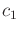,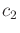,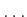, and would like to incrementally compute their average. You are not told the total number of samples in advance, and at any point you are required to report the current average. Let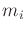denote the average of the firstsamples,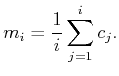(10.80)

To efficiently computefrom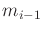, multiplyby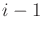to recover the total, add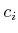, and then divide by: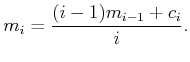(10.81)

This can be manipulated into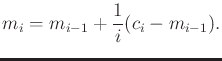(10.82)

Now consider the problem of estimating the expected cost-to-go,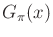, at every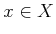for some fixed plan,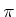. If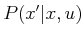and the costs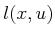were known, then it could be computed by solving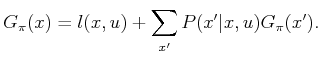(10.83)

However, without this information, we must rely on the simulator.

From each, suppose that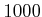trials are conducted, and the resulting costs to get to the goal are recorded and averaged. Each trial is an iterative process in whichselects the action, and the simulator indicates the next state and its incremental cost. Once the goal state is reached, the costs are totaled to yield the measured cost-to-go for that trial (this assumes that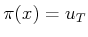for all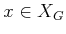). Ifdenotes this total cost at trial, then the average,, overtrials provides an estimate of. Astends to infinity, we expectto converge to. The update formula (10.83) can be conveniently used to maintain the improving sequence of cost-to-go estimates. Let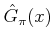denote the current estimate of. The update formula based on (10.83) can be expressed as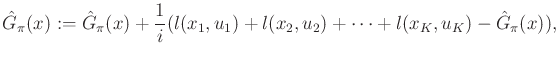(10.84)

in which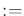means assignment, in the sense used in some programming languages.

It turns out that a single trial can actually yield update values for multiple states [930,96]. Suppose that a trial is performed fromthat results in the sequence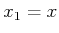,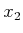,,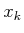,,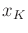,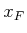of visited states. For every state,, in the sequence, a cost-to-go value can be measured by recording the cost that was accumulated fromto: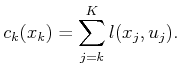(10.85)

It is much more efficient to make use of (10.85) on every state that is visited along the path.

Subsections
Steven M LaValle 2020-08-14